Next: Current Conservation Up: Dirac Equation Previous: Dirac Equation

# Wave Equation for a Spin-1/2 Particle

We seek a relativistic covariant equation of the form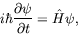(5.1)

which is first order in the time derivative and will have positive definite probability density. Assuming such an equation is also linear in the space derivatives, we can write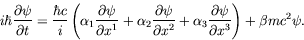(5.2)

Using operator notation we have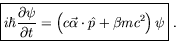(5.3)

For invariance under spatial rotation, the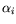can not be numbers and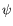can not be a scalar. In analogy with the spin wave function of nonrelativistic quantum mechanics, we chooseto be a vector, andand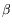to be matrices. Explicitly,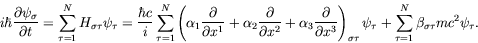(5.4)

We thus have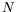coupled first-order equations.

These equations must

1. have free-particle solutions that satisfy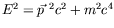,
2. yield a continuity equation and probability interpretation of, and
3. be Lorentz covariant.

For the first condition to be satisfied, each component of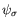must satisfy the Klein-Gordon equation. Applying the operator (5.1) twice gives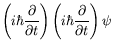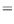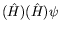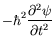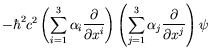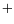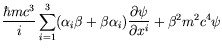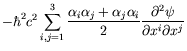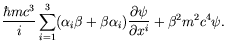(5.5)

To obtain the Klein-Gordon equation the following must be satisfied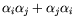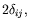(5.6)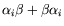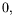(5.7)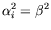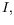(5.8)

where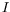represents an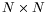unit matrix. We will not write the unit matrix explicitely in the wave equation unless it is required for clarity. This should not create any confusion since matrices can only equal matrices.

No terms in the Hamiltonian can have any space or time coordinates. Such terms would have the property of space-time dependent energies and would give rise to forces. Space and time derivatives can only appear in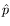and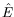, but not inand, since the equation is to be linear in these derivatives. Thusandare independent of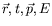and hence commute with them.

Since the Hamiltonian must be hermitian,andmust be hermitian matrices. Since the matrices are hermitian they must be square.

Sinceandanticommute according to equation 5.7, they are traceless. This can be seen as follows: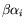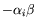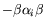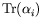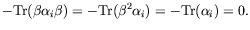(5.9)

where the last line follows from the cyclic property of the trace. The choice of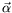andis not unique. All matrices related to these by any unitarymatrix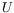(which preserves the anti-commutation relations) are allowed: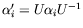and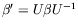.

Since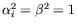, the eigenvalues ofandare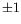. Since the trace is the sum of eigenvaluesand, must be of even dimensions. For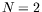, only three anti-commuting matrices exist (the Pauli matrices). Thus the smallest dimension allowed is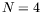.

If one matrix is diagonal, the others can not be diagonal or they would commute with the diagonal matrix. We can write a representation that is hermitian, traceless, and has eigenvalues of: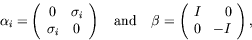(5.10)

where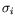are the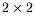Pauli matrices andis theunit matrix.Next: Current Conservation Up: Dirac Equation Previous: Dirac Equation
Douglas M. Gingrich (gingrich@ ualberta.ca)
2004-03-18